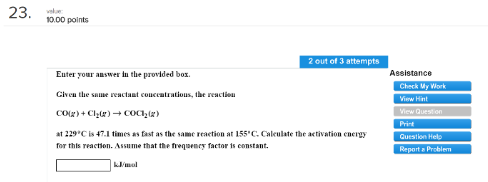# Problem: Enter your answer in the provided box.Given the same reactant concentrations the reaction CO (g) + Cl2 (g) → COCl2 (g) at 229°C is 47.1 times as fast as the same reaction at 155°C. Calculate the activation energy for this reaction. Assume that the frequency factor is constant.

###### FREE Expert Solution
85% (292 ratings)###### Problem Details

Given the same reactant concentrations the reaction

CO (g) + Cl2 (g) → COCl2 (g)

at 229°C is 47.1 times as fast as the same reaction at 155°C. Calculate the activation energy for this reaction. Assume that the frequency factor is constant.